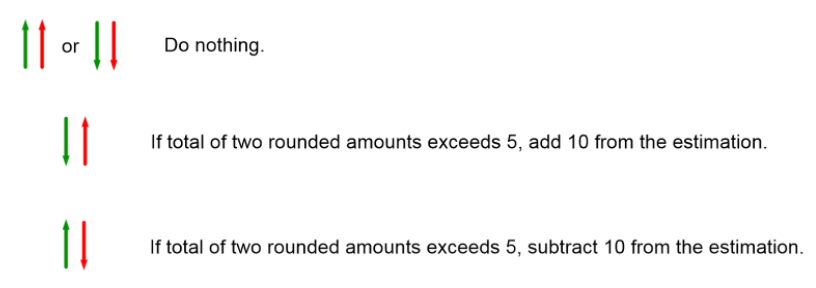Smartick is a fun way to learn math!Jul24

# Round and Estimate Subtraction Problems

In this post, we are going to learn how to round and use estimation in subtraction problems. Mastering this skill is helpful in verifying that an exact answer is of the correct order of magnitude and also in determining whether an answer is reasonable or not.

### Round and Estimating Subtraction Problems in Tens

1. Round the numbers that numbers that will be subtracted to the nearest ten: if the number ends in 0, 1, 2, 3 or 4, we round down. If the number ends in 5, 6, 7, 8 or 9, we round up
2. Subtract the rounded numbers.
3. Even out the amount that was rounded. The rounded quantity is the number that we’ve lessened or added to the number in order to round.
• If both numbers were rounded up or down, we don’t have to do anything more
• If the first number was rounded down and the second was rounded up, and the total of the rounded amounts is 5 or greater, we have to add 10 to the estimation.
• If the first number was rounded up and the second down, and the total of the rounded amounts is 5 or greater, we have to subtract 10 from the estimation.Example: 65 – 32

1. Round the two numbers:

65 –> 70

32 –> 30

1. Subtract the rounded numbers:

70 – 30 = 40

1. Even out the rounded numbers:

65 –> 70        32 –> 30The first number was rounded up and the second was rounded down, so we need to even out the rounded quantity:

65 –> 70        32 –> 305                          2

5 + 2 = 7

The sum of the rounded amounts turns out to be greater than 5, so we need to subtract 10 from the estimation:

40 – 10 = 30

The correct estimation then, is 30.

### Round and Estimating subtraction problems in hundreds

1. Round the numbers that numbers that will be subtracted to the nearest hundred.
2. Subtract the rounded numbers.
3. Even out the amount that was rounded.
• If both numbers were rounded up or down, we don’t have to do anything more
• If the first number was rounded down and the second up, and the total of the rounded amounts is 50 or greater, we have to add 100 to the estimation.
• If the first number was rounded up and the second down, and the total of the rounded amounts is 5 or greater, we have to subtract 100 from the estimation.

Example: 658 – 171

1. Round the two numbers:

658 –> 700

171 –> 200

1. Subtract the rounded numbers:

700 – 200 = 500

1. Even out the rounded quantity:

658 –> 700       171 –> 200Both numbers were rounded up so you don’t need to change anything; the correct estimation is 500.

I hope you liked this post! If you want to keep learning, you can try Smartick’s free trial.